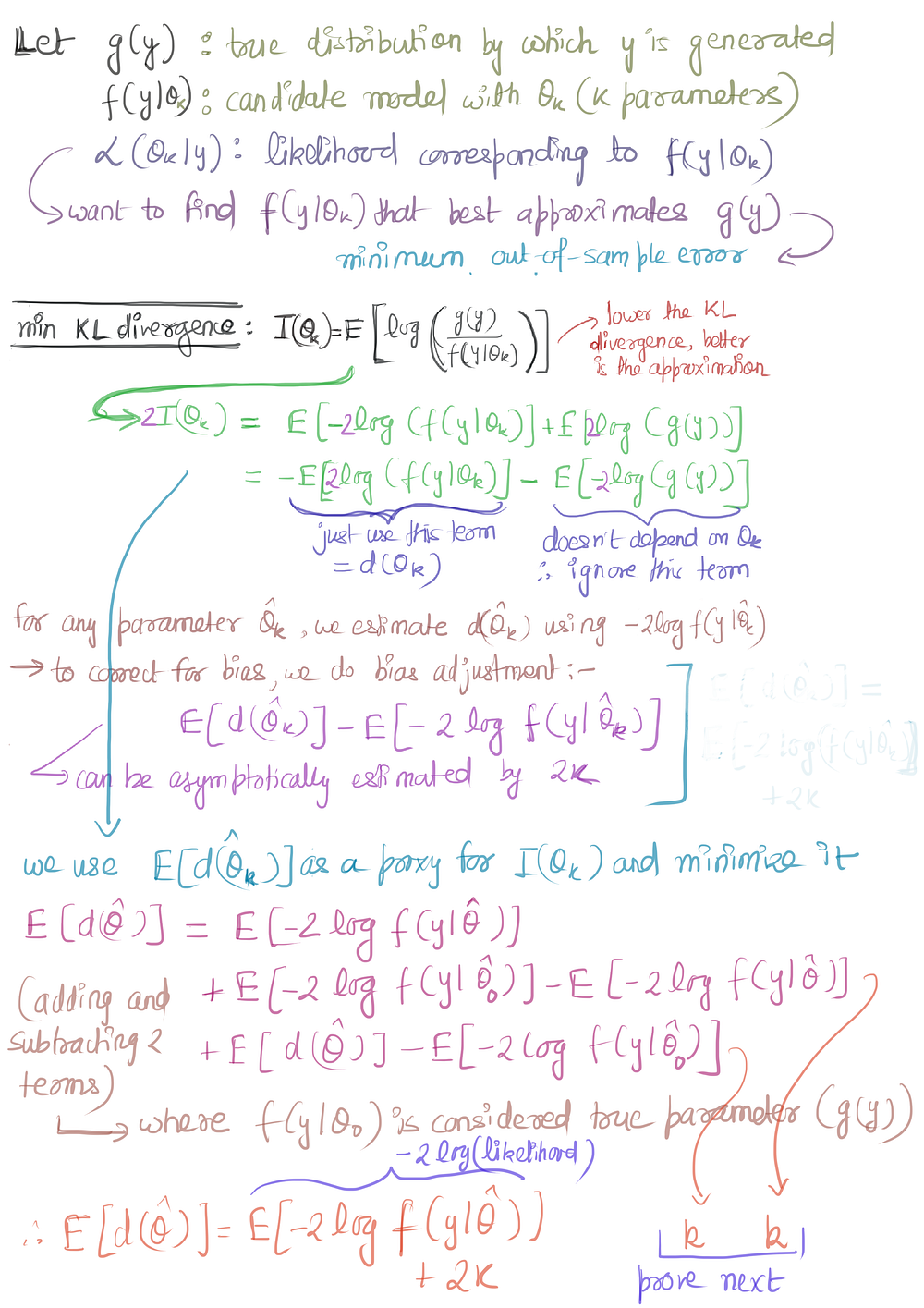# Akaike Information Criteria

Original article can be found here (source): Artificial Intelligence on Medium# Akaike Information Theory

## The idea behind AIC

All of us have used AIC for model selection. This blog is about the idea behind AIC, what is it and why is it used for model selection. While we have been told how to calculate AIC, at least I was never taught the logic behind why are we doing this — this blog is to cover that.

AIC is an estimate for the out-of-sample error. AIC is based on information theory. He called it an entropy maximization principle and minimizing AIC is equivalent to maximizing entropy in a thermodynamic system. Thus, in the language of information theory, we can say that while coding a model (where we can never find the exact model), some information is lost in representing the process by which the data was generated.

AIC measures the relative loss of information. Since we do not the exact model, we cannot measure the exact loss. Thus we measure relative loss among the different models (from which we have to select our model). If we have 3 models with AIC values 100, 102, and 110, then the second model is exp((100 − 102)/2) = 0.368 times as probable as the first model to minimize the information loss. Similarly, the third model is exp((100 − 110)/2) = 0.007 times as probable as the first model to minimize information loss.

AIC is given by 2 x number of parameters — 2 log(Likelihood)

When selecting the model (for example polynomial function), we select the model with the minimum AIC value. Or if we can select the top 2–3 models, collect more data and select the once with minimum AIC. This blog is about — where does this formula of AIC come from?

In AIC, we try to minimize the (proxy of) KL divergence between the model and the ground truth function. AIC is the calculation for the estimate of the proxy function. Thus minimizing the AIC is akin to minimizing the KL divergence from the ground truth — hence minimizing the out of sample error. The derivation for AIC is shown in the following two images.

Bayesian Information Criteria (BIC) is calculated similarly to AIC. Instead of 2k, BIC uses 2 ln(n)k. These are called the penalty terms. It is argued that if the true model is present in the set of models, BIC selects the true model with probability 1, given n tends to infinity. Since we never really have the true model in the set of candidate models, this property is not highly regarded. Also, AIC minimizes the risk of selecting a very bad model.

Reference